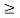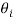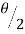# Atomic Force Microscope (AFM)

2486 words (10 pages) Essay

1st Feb 2018 Physics Reference this

Tags:

Disclaimer: This work has been submitted by a university student. This is not an example of the work produced by our Essay Writing Service. You can view samples of our professional work here.

Any opinions, findings, conclusions or recommendations expressed in this material are those of the authors and do not necessarily reflect the views of UKEssays.com.

Atomic force microscope (AFM) was invented by Gerd Binnig, Calvin F. Quate and Christopher Herber in 1986 . AFM relies on interactive force to generate an image. Interactive force occurs between a tip and sample surface using the probe which is a micro-fabricated elastic cantilever with a sharp tip on the end.

Cantilevers are generally made from silicon (Si) or silicon nitride (Si3N4) materials . The deflection of the AFM cantilever can be obtained by using Hooke’s Law ;

where ΔZ is the deflection of cantilever (Figure 34) which is determined by divided the acting force F with spring constant k.

The optical detection system of the AFM detects the displacement of the cantilever. This system consists of a four-quadrant photodiode and a laser source. In simple terms, laser beam is focused on the back of the cantilever and reflected here and collected in a photodiode. Each section of the photodiode creates photocurrents. Through this optical detection system, the attractive or repulsive forces due to the tip bending or cantilever torsion due to the lateral component of tip-sample interaction can be examined. Whether the reference values in the photodiode sections are indicated as I01, I02, I03, I04 and I1, I2, I3, I4 are the current values, the variation of currents from different sections of the photodiode ΔIi = Ii – I0i can be characterized with ΔIZ = [(I1 + I2) – (I3 + I4)] and ΔIL = [(I1 + I4) – (I2 + I3)] for deflection and torsion of cantilever.

In feedback mode, the ΔIZ value is used as an input signal and output signal adjusts the Z position of the scanner. The main purpose of the feedback system is to keep the tip-sample interval (ΔZ) constant. If ΔZ = constant mode is used, tip moves along the sample surface. Accordingly, Z = f(x,y) surface topography can be acquired with respect to applied voltage on the Z-electrode of the scanner (Figure 35).

The interactive forces which are mentioned before can be explained by considering van der Waals forces . Two atoms are located at a distance r from each other, the van der Waals potential energy of these two atoms is approximated by the exponential function which is known as Lennard-Jones potential .

where the first term describes the attraction of long distances due to dipole-dipole interaction and second term describes short range repulsion caused by the Pauli exclusion principle. The r0 parameter is the equilibrium distance between two atoms and the energy value in the minimum (Figure 36).

Distance between the tip and the sample is dependent van der Waals force which can be seen in Figure 37.

The main AFM scan modes are divided into three parts: contact mode, tapping (semi-contact) mode and non-contact mode. In our AFM measurements, always tapping mode is used for characterizing surface.

Scanning electron microscope (SEM) was invented by Max Knoll in 1935 as a tool for surface characterization . SEM is a type of electron microscope that creates images of a sample by using focused beam of electrons and gain information about surface structure and composition. The results of interaction between electron beams and the sample can be seen in Figure 38.

The types of signals produced by a collision between sample and focused electron beam contain auger electrons, secondary electrons, back-scattered electrons and characteristic x-rays (Figure 38). Depth ranges of the interaction volumes are investigated with respect to various types of scattered electrons and x-rays. Auger electrons have ~ 1 nm, secondary electrons have ~ 100 nm, back-scattered electrons have ~ 1-2 µm and X-rays have ~ 5 µm depth ranges [89-91].

SEM utilizes vacuum conditions and uses electrons to form an image. All water must be removed from the sample because the water would vaporize in the vacuum. Metal, semi-metal and semiconductor samples are conductive and no preparation required before being used. All non-metals need to be made conductive by covering the sample with a thin layer of conductive material by using sputter coater.

SEM consists of an electron gun which produces a beam of electrons. The electron beam follows a vertical path through the microscope, which is held in a vacuum. The beam travels through electromagnetic fields and lenses which focus the beam down toward the sample. When the beam hits the sample, electrons and X-rays are ejected from the sample. Detectors collect these X-rays, backscattered electrons and secondary electrons and then convert them into a signal that is sent to a screen. This produces the final image (Figure 39).

For the topographic images, we use a secondary electron detector because; secondary electrons are closer to the sample surface. Backscattered electron detector gives knowledge due to the atomic contrast. Elements of higher atomic number give a brighter image (dark-bright contrast). For the unknown elements, x-ray detector (EDX) is used. This detector collects the x-rays which are scattered from the sample surface. Each element has a different x-ray diffractometers. The difference between XRD and EDX is that XRD for the crystal composition and uses the x-rays. However; EDX gives information for the elemental composition by using electrons.

Differential Interference Contrast (DIC) or also known as Nomarski microscopy was invented by George Nomarski in 1960 . DIC is a type of optical characterization technique which involves Wollaston (Nomarski) prisms for separating and recombined a polarized light. Polarized light is formed when light from lamp source is passed through a polarizer.

Working principle of the DIC microscope is based on the polarized light source which is firstly divided into two rays (ordinary and extra-ordinary) by first condenser (Wollaston or Nomarski) prism . These two rays are vibrating perpendicular with each other. These two rays reach the condenser and lengthen parallel to each other. Distance between these two parallel rays is slightly equal to the optical path difference. Perpendicular vibration between rays does not occur to interference. Later, two rays passed through the sample and wave lengths of these rays changes with respect to the sample properties such as thickness or refractive indices. Two parallel rays go into the objective and begin reunification. Second Wollaston or Nomarski prism is recombined two rays totally. Analyzer prevents the interference of the rays. The final image which can be seen with eyepieces has the three-dimensional appearance of the sample. This pseudo three-dimensional effect results from the shadow impact that involves the brighter and darker sides. Working principle of Nomarski microscope can be seen clearly in Figure 40.

Reflected high energy electron diffraction (RHEED) is an in-situ characterization technique which gives knowledge about surface epitaxial changes during the growth. To understand RHEED geometry, some theoretical background of the electron diffraction and kinematic scattering will be given in this section. Theoretical explanations can be started with the relativistic electron energy relation in terms of momentum. For high energetic electrons (E50 keV), relativistic effect should be taken into account .

Acceleration voltage, electron rest mass, electron momentum and speed of light are indicated with V0, m0, p and c0, respectively. If we rewrite this above equation leaving the momentum alone;

Due to the wave-particle duality, a beam of electrons can be diffracted just like a beam of light or a matter wave. Louis de Broglie proposed particles to behave like a wave . Therefore, electrons wave-particle property can be explained by;

Equation 2 can be substitute into the equation 3,

Definition of “c” is speed of light and accepted value is 3×108 m/s. “h” is Planck’s constant and is equal to 4.14×10-15 eV.s. Rest mass of electron is indicated with “m0” and it is equal to 0.51×106 eV/c2. When these numeric values are substituted in the de Broglie relation, wave length equality becomes;

If the energy value of the incident beam is equal to 30 keV, wavelength is equal to 0.07 Å according to the above equation. RHEED patterns, as seen on the phosphorescent screen, are the result of the constructive interference of the scattered wave. Constructive interference term is related to the Bragg condition which is explained by ;

As can be seen in Figure 42; the incidence angleis equal tofor elastic scattering process. Also in this figure, scattering or momentum transfer vector representation can be understood.

Under the conditions of elastic scattering, incidence and scattered wave vectors are â”‚kËˆâ”‚=â”‚kâ”‚= 2π/λ . Diffraction maximum occurs when the Laue condition is satisfied and this condition is ;

Order of diffraction is demonstrate by n. RHEED patterns on the phosphorescent screen are reflection of the surface atoms in reciprocal lattice space. If the real space basis vectors indicate as a, b and c, reciprocal basis vectors become a*, b* and c*. In addition, the relation between real and reciprocal space basis vectors is ;

Translation vector is also indicated for reciprocal space as;

h, k and l are miller indices. Laue condition under the constructive interference for certain miller indices is s = G .

The incident electron beam hit the crystal surface which is growing epitaxially at low angle of incidence and is reflected onto the phosphorescent screen to form RHEED patterns (Figure 43). RHEED patterns include spots, streaks, rings and lines.

The intensity oscillation changes of the RHEED spots on the screen give information about growth parameters such as removal of oxides from epi-ready substrates surface, surface roughness of the grown layers and crystal quality of the layers . When the incident beam electrons reach the epi-ready surface at the beginning of the growth, incident electrons get through minimum diffraction because of surface smoothness. Therefore, RHEED patterns have maximum intensity. When a layer nucleates on the surface, electrons get through maximum diffraction and this condition led to minimum intensity of the RHEED patterns.

Calculation of the lattice constant for growth material from the RHEED images and the percentage of the error between the accepted and calculated value of the lattice parameters will be discussed in Chapter 4.

Raman spectroscopy was discovered by C. V. Raman and K. S. Krishnan in 1928 . In addition, C. V. Raman was awarded the Nobel Prize for discovery of Raman in 1930 . Raman spectroscopy can be used for distribution of vibrational modes to generate like a chemical maps. It is possible to combine Raman spectroscopy with hardware system. The data signal collected by the detector and then sent to the hardware system for analysis. In order to investigate the effects of wet chemical etching procedures on especially Te, CdTe and GaAs vibration modes in this study, two dimensional (x, y) maps were recorded by Raman spectroscopy at room temperature. Raman measurements were performed by a confocal Raman system.

Laser beam comes from the laser source and passes through the filters. Beam splitter deflects a portion of light onto the optical microscope. Light is passed through a proper objective and laser light is focused onto the sample. Sample stands on the XYZ stage. Laser light is scattered from the sample and follows a proper optical path to reach a detector. Computer system is used to analyze signals which are collected by detector (Figure 44).

## Related Services

View all

### DMCA / Removal Request

If you are the original writer of this essay and no longer wish to have your work published on the UKDiss.com website then please:

Related Services